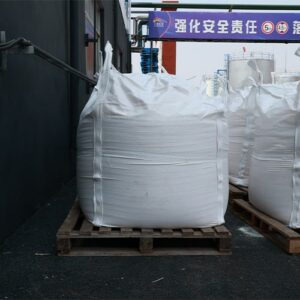# Deciphering the Molar Mass of Sodium Formate: Unveiling Molecular Complexity

## Giới thiệu

In the realm of chemistry, understanding the composition and structure of molecules is paramount. Sodium formate, a versatile chemical compound, holds intrigue not only for its properties but also for its molar mass—a fundamental value that plays a pivotal role in various calculations. This article embarks on a journey to unravel the complexities of sodium formate’s molar mass, delving into its molecular makeup, significance, and applications in the realm of quantitative chemistry.

• Giới thiệu
• Molar Mass: The Molecular Identity Card
• Dạng muối natri‘s Molecular Composition
• Calculating Molar Mass
• Atomic Mass Units (amu)
• Sum of Atomic Weights
• Significance of Molar Mass
• Stoichiometry and Chemical Reactions
• Dilution and Concentration Calculations
• Applications Beyond Calculation
• Analytical Chemistry
• Synthetic Chemistry
• FAQ
• Sự kết luận

## Molar Mass: The Molecular Identity Card

Molar mass serves as the molecular identity card—a numerical representation of a compound’s mass in atomic mass units (amu). It is a cornerstone of quantitative chemistry, influencing a spectrum of calculations and analyses.

## Dạng muối natri‘s Molecular Composition

Sodium formate, with the chemical formula NaCHO2, consists of sodium ions (Na+) and formate ions (CHO2-). This molecular makeup forms the foundation for calculating its molar mass.

## Calculating Molar Mass### Atomic Mass Units (amu)

Atomic mass units (amu) are the standard unit of measurement for molar mass. It is defined as one-twelfth the mass of a carbon-12 atom. The molar mass of a compound is the sum of the masses of its constituent atoms, each multiplied by its respective subscript.

### Sum of Atomic Weights

To calculate the molar mass of sodium formate, one must add the atomic masses of its constituent elements: sodium (Na), carbon (C), hydrogen (H), and oxygen (O). The atomic masses can be found on the periodic table and are often expressed in atomic mass units (amu).

## Significance of Molar Mass

### Stoichiometry and Chemical Reactions

Molar mass is integral to stoichiometry—the study of quantitative relationships in chemical reactions. It serves as a bridge between the macroscopic world (grams) and the atomic world (moles), enabling the determination of reactant and product quantities.

### Dilution and Concentration Calculations

In solution chemistry, molar mass aids in dilution and concentration calculations. By knowing the molar mass of a solute, scientists can accurately prepare solutions of specific concentrations, contributing to accurate experimental outcomes.

## Applications Beyond Calculation### Analytical Chemistry

Molar mass plays a role in analytical techniques such as mass spectrometry, where it aids in identifying compounds by comparing their molar masses to reference values.

### Synthetic Chemistry

In synthetic chemistry, molar mass informs reaction conditions and stoichiometric ratios. It guides the design of reactions and the precise addition of reagents for desired outcomes.

## FAQ

Q1: Is the molar mass of Dạng muối natri constant, regardless of its form?
A1: Yes, the molar mass of sodium formate remains constant regardless of its physical state or form, as it is determined by the atomic composition of its elements.

Q2: How does molar mass relate to molecular weight?
A2: Molar mass and molecular weight are often used interchangeably. They both refer to the mass of a substance in atomic mass units (amu), although molar mass specifically refers to the mass of one mole of a substance.

Q3: Can molar mass be used to determine the empirical formula of a compound?
A3: Yes, molar mass can be used to determine the empirical formula by comparing the molar mass of the compound to the calculated molar mass based on its empirical formula.

## Sự kết luận

Sodium formate’s molar mass, a numerical embodiment of its molecular composition, holds significance that extends beyond numerical calculations. It shapes our understanding of chemical reactions, guides analytical techniques, and influences synthetic endeavors. As chemistry evolves, the molar mass of compounds like sodium formate continues to be a cornerstone, underscoring the intricate relationship between molecular properties and quantitative measurements.